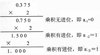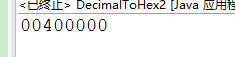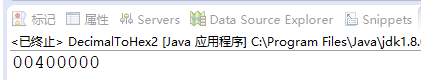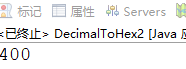• Python实现的进制转换器#1、十六进制以内实数任意进制对任意进制的转换 #2、小数位数超过十位保留十位小数 #3、包含负数的转换（是数值的转换而不是补码） #4、能够实现更高进制的转换：直接增加waitNumber中的字母...
• 二进制数组转换为二进制字符串 将二进制数组转换为十六进制字符串 将十六进制转换为二进制字节数组 将十六进制转换为二进制字符串
• 纯JavaScript编写的各种常见进制转换器，例如：二进制转换为八进制，八进制转十六进制，此转换器都能实现！js
• 主要介绍了C语言用栈实现十进制转换为二进制的方法,结合实例形式分析了C语言栈的定义及进制转换使用技巧,需要的朋友可以参考下
• 好用的浮点数与二进制转换工具,通讯开发必备实用小工具，4字节浮点数转换，支持正序倒序转换。浮点数转二进制，二进制转浮点数，转换准确。
• C语言实现十进制向任意进制转换代码，已经经过调试无问题。可以直接使用C语言
• QT编写的进制转换工具，包含代码，可以实现32位二进制与十进制，16进制相互转换，可以对二进制单独位修改Qt
• 利用栈实现进制转换，支持16进制以下进制（2-10、16），更改相应的参数还可扩展其他进制转换，还可以通过更改宏定义来扩宽进制转换的数据范围。用户通过输入想要转换的进制和待转换的数字
• 包含ASIIC、16进制，数值，字符串互转；二进制，十六进制互转；十进制显示的十六进制格式字符串转Labview所识别的十六进制，通讯下位机必备技能。总共8个VI！
• 西门子TIA博途进制转换功能pdf,西门子TIA博途进制转换功能
• C语言实现十进制转换成二八十六进制的转换，代码已经经过调试可以使用，放心下载！C语言
• 适用于将二进制转换为十进制，A为十进制，B为二进制。{A,B}每次左移一位，判断A的每四位是否＞4，若大于四则+3，否则保持不变；B为多少位二进制数则左移多少次。最终A是B转换成十进制的数。代码为32位二进制转换...
• C语言-顺序栈实现十进制转换为二进制-八进制-十六进制
• 数据结构进制转换数据结构进制转换数据结构进制转换数据结构进制转换数据结构进制转换数据结构进制转换
• 可以将ANSI和UNICODE字符串转换成16进制数据，也可以将16进制数据转换成ANSI和UNICODE字符串，UNICODE字符串还可以选择高位在前还是低位在前，非常的好用。
• 进制转换 在生活和实际开发中，进制转换是一个常考的知识点。 虽然Java开发者已经对其进行了封装，但还是要学会自己编写相应的进制转换器的。 常用的进制有二进制，八进制，十进制，十六进制。 二.将整数转换成十六...
一. 进制转换
在生活和实际开发中，进制转换是一个常考的知识点。 虽然Java开发者已经对其进行了封装，但还是要学会自己编写相应的进制转换器的。 常用的进制有二进制，八进制，十进制，十六进制。
二.将整数转换成十六进制
二.一 将int整数转换成十六进制（一）
先写一个主方法，接下来，所有的方法都是通过main 方法来调用的。 不用Junit测试。 做静态的方法，类似于工具的形式。
public static void main(String []args){
System.out.println(printOX1(1024));
}

先用最简单的方法进行写程序。 求十六进制，就是将这个数字不断的除以16，然后得到余数。 去判断这个余数的大小，如果是0 ~ 9 ，那么就用数字0 ~ 9 来表示，如果是10 ~ 15 ,那么 就用字符’A’ ~ ‘F’ 或者小写的 ‘a’ ~ ‘f’ 来表示。 然后，用商来替换这个数字，继续进行循环。 最简单的方式，先用一个字符串来接收转换后的进制结果。要循环八次。 为什么用8呢，因为int型是4个字节，十六进制最大用四个字符来表示。 所以，最大是4*8/4=8 个长度。
public static String printOX1(int num){
//1. 判断，如果是0的话，就直接返回0
if(num==0){
return "0";
}
//2.16进制的话，存储int型，可以存储4*32/16=8 个长度。
StringBuilder sb=new StringBuilder();
for (int i = 0; i <8; i++) {
//3. 将这些数字除以16,得到余数。 求的是低四位。
int temp=num&15;
if(temp>9){
sb.append(temp-10+'A');
}else{
sb.append(temp);
}
num=num>>>4; //除以16,得到商。 将商当成除数。
}
return sb.toString();
}

上面这个运行输出后，结果是:这个顺序是颠倒的，并且还有多余的0存在。
二.二 将int整数转换成十六进制（二）
改进: 根据余数来求数字和字符，其实是可以转换成数组的。 利用数组的查表法进行相应的改变。 将余数放入到数组的下标索引上，就可以取出相应的表示字符。
public static String printOX2(int num){
//1. 判断，如果是0的话，就直接返回0
if(num==0){
return "0";
}
// 定义一个字符数组，这个索引与十六进制的表示方式一样。
char [] hexs={'0','1','2','3','4','5','6','7','8','9',
'A','B','C','D','E','F'};
StringBuilder sb=new StringBuilder();
for (int i = 0; i <8; i++) {
int temp=num&15;
sb.append(hexs[temp]); // 根据下标去求数组中查询相应的值。
num=num>>>4;
}
return sb.toString();
}

运行后，结果仍然是: 00400000, 顺序颠倒，有零。二.三 将int整数转换成十六进制（三）
利用数组的方式去解决。 将得到的这个值，倒序放入到一个数组中，就是将004 00000 倒序放入数组中。 那么 此时 a=0,a=0,… … a=0, a=4, a=0,a=0; 然后将这个数组从非零的索引位置处进行输出即可。 要记录下这个非零的索引位置。
public static String printOX3(int num){
//1. 判断，如果是0的话，就直接返回0
if(num==0){
return "0";
}
//2.16进制的话，存储int型，可以存储4*32/16=8 个长度。
char [] hexs={'0','1','2','3','4','5','6','7','8','9',
'A','B','C','D','E','F'};
char [] tabs=new char;
StringBuilder sb=new StringBuilder();
int pos=tabs.length;
while (num>0) {  //不知道要运行几次。
//3. 将这些数字除以16,得到那个要转换的值。
int temp=num&15;
tabs[--pos]=hexs[temp];  //上面用的是长度，这里用--pos. 要注意，索引越界的问题。
num=num>>>4;
}
for(int i=pos;i<8;i++){
sb.append(tabs[i]);
}
return sb.toString();
}

运行之后，就是:正确的十六进制数字。
二.四 将int整数转换成十六进制（四）
利用String 的format 格式化方法进行求解。 格式信息是 %x. x为十六进制。
    String.format("%x",1024);   // 是String 类型

二.五 将int整数转换成十六进制（五）
Java底层开发人员，封装了一个方法。 可以自动将数字进行转换成十六进制。 开发中，推荐使用这一个。
	Integer.toHexString(1024)    //转换成十六进制。 二进制，八进制，也有类似的方法。

三. 十进制转其他的进制
只支持常见的二进制，八进制，十六进制。 这种方式，暂时不支持其他的进制。后面有其他的方法会慢慢支持的。
public static void main(String []args){
int num=1024;
System.out.println("十六进制:"+toHex(num)); //转换成十六进制
System.out.println("二进制:"+toBinary(num)); //转换成二进制
System.out.println("八进制:"+toOct(num)); //转换成八进制
}

其中，十六进制的方法是:
public static String toHex(int num) {
return  toTrave(num,15,4); //基数是15，右移4位。
}

虽然二进制和八进制，已经可以证明，肯定是数字型的。但由于位数相比较十进制较多，用int 或者long接收的话，都有可能造成溢出。 所以，不建议用long 或者int 接收 。 直接用字符串接收，是数字型的字符串。 其中，八进制的方法是:
public static String toOct(int num) {
}

其中，二进制的方法是:
public static String toBinary(int num) {
}

共同的方法是 toTrave()的定义是:
public static String toTrave(int num,int base,int moveIndex){
//1. 判断，如果是0的话，就直接返回0
if(num==0){
return "0";
}
//2.16进制的话，存储int型，可以存储4*32/16=8 个长度。
char [] hexs={'0','1','2','3','4','5','6','7','8','9',
'A','B','C','D','E','F'};
char [] tabs=new char;
StringBuilder sb=new StringBuilder();
int pos=tabs.length;
while (num>0) {  //不知道要运行几次。
//3. 将这些数字除以基数,得到那个要转换的值。
int temp=num&base;
tabs[--pos]=hexs[temp];  //上面用的是长度，这里用--pos. 要注意，索引越界的问题。
num=num>>>moveIndex;
}
for(int i=pos;i<8;i++){
sb.append(tabs[i]);
}
return sb.toString();
}

与上面的printOX3（） 方法是一样的。 只是将15，和>>>4 的4 转换成局部变量而已。
四. String.format 方法的支持
		System.out.println(String.format("%x",1024));//十六进制
System.out.println(String.format("%o",1024)); //八进制
System.out.println(String.format("%d",1024)); //十进制
System.out.println(String.format("%b",1024)); //%b,并不是二进制，而是会输出true. 非空为true

五. Integer 的toString() 方法封装
		System.out.println(Integer.toBinaryString(1024)); //二进制
System.out.println(Integer.toOctalString(1024)); //八进制
System.out.println(Integer.toHexString(1024)); //十六进制

如果要转换成其他进制的话，用toString(十进制数字num,要转换的进制位) 的方法。
System.out.println(Integer.toString(1024,7));   //转换成七进制数
System.out.println(Integer.toString(1024,2));  //当然，也可以用它转换成二进制数。

五. Integer 的parseInt(“数字字符串”,“原先的进制”) 和valueOf(“数字字符串”,原先的进制) 方法封装
这两个方法，都是转换成十进制的。
System.out.println(Integer.parseInt("400",16)); //将16进制的400转换成十进制
System.out.println(Integer.valueOf("400",8));//将8进制的400转换成十进制

谢谢!!
展开全文• 浮点数转十六进制转换器，包含32位、64位浮点数的转换和10进制到16进制的转换。
• 进制转换：二进制、八进制、十六进制、十进制之间的转换 不同进制之间的转换在编程中经常会用到，尤其是C语言。 将二进制、八进制、十六进制转换为十进制 二进制、八进制和十六进制向十进制转换都非常容易，就是...
进制转换：二进制、八进制、十六进制、十进制之间的转换
不同进制之间的转换在编程中经常会用到，尤其是C语言。
将二进制、八进制、十六进制转换为十进制
二进制、八进制和十六进制向十进制转换都非常容易，就是“按权相加”。所谓“权”，也即“位权”。
假设当前数字是N进制，那么：
对于整数部分，从右往左看，第i位的位权等于Ni-1
对于小数部分，恰好相反，要从左往右看，第j位的位权为N-j。
更加通俗的理解是，假设一个多位数（由多个数字组成的数）某位上的数字是1，那么它所表示的数值大小就是该位的位权。
1) 整数部分
例如，将八进制数字53627转换成十进制：
53627 = 5×84 + 3×83 + 6×82 + 2×81 + 7×80 = 22423（十进制）
从右往左看，第1位的位权为 80=1，第2位的位权为 81=8，第3位的位权为 82=64，第4位的位权为 83=512，第5位的位权为 84=4096 …… 第n位的位权就为 8n-1。将各个位的数字乘以位权，然后再相加，就得到了十进制形式。
注意，这里我们需要以十进制形式来表示位权。
再如，将十六进制数字9FA8C转换成十进制：
9FA8C = 9×164 + 15×163 + 10×162 + 8×161 + 12×160 = 653964（十进制）
从右往左看，第1位的位权为160=1，第2位的位权为 161=16，第3位的位权为 162=256，第4位的位权为 163=4096，第5位的位权为 164=65536 …… 第n位的位权就为16n-1。将各个位的数字乘以位权，然后再相加，就得到了十进制形式。
将二进制数字转换成十进制也是类似的道理：
11010 = 1×24 + 1×23 + 0×22 + 1×21 + 0×20 = 26（十进制）
从右往左看，第1位的位权为20=1，第2位的位权为21=2，第3位的位权为22=4，第4位的位权为23=8，第5位的位权为24=16 …… 第n位的位权就为2n-1。将各个位的数字乘以位权，然后再相加，就得到了十进制形式。
2) 小数部分
例如，将八进制数字423.5176转换成十进制：
423.5176 = 4×82 + 2×81 + 3×80 + 5×8-1 + 1×8-2 + 7×8-3 + 6×8-4 = 275.65576171875（十进制）
小数部分和整数部分相反，要从左往右看，第1位的位权为 8-1=1/8，第2位的位权为 8-2=1/64，第3位的位权为 8-3=1/512，第4位的位权为 8-4=1/4096 …… 第m位的位权就为 8-m。
再如，将二进制数字 1010.1101 转换成十进制：
1010.1101 = 1×23 + 0×22 + 1×21 + 0×20 + 1×2-1 + 1×2-2 + 0×2-3 + 1×2-4 = 10.8125（十进制）
小数部分和整数部分相反，要从左往右看，第1位的位权为 2-1=1/2，第2位的位权为 2-2=1/4，第3位的位权为 2-3=1/8，第4位的位权为 2-4=1/16 …… 第m位的位权就为 2-m。
更多转换成十进制的例子：
二进制：1001 = 1×23 + 0×22 + 0×21 + 1×20 = 8 + 0 + 0 + 1 = 9（十进制）
二进制：101.1001 = 1×22 + 0×21 + 1×20 + 1×2-1 + 0×2-2 + 0×2-3 + 1×2-4 = 4 + 0 + 1 + 0.5 + 0 + 0 + 0.0625 = 5.5625（十进制）
八进制：302 = 3×82 + 0×81 + 2×80 = 192 + 0 + 2 = 194（十进制）
八进制：302.46 = 3×82 + 0×81 + 2×80 + 4×8-1 + 6×8-2 = 192 + 0 + 2 + 0.5 + 0.09375= 194.59375（十进制）
十六进制：EA7 = 14×162 + 10×161 + 7×160 = 3751（十进制）
将十进制转换为二进制、八进制、十六进制
将十进制转换为其它进制时比较复杂，整数部分和小数部分的算法不一样，下面我们分别讲解。
1) 整数部分
十进制整数转换为N进制整数采用“除N取余，逆序排列”法。具体做法是：
将N作为除数，用十进制整数除以N，可以得到一个商和余数；
保留余数，用商继续除以N，又得到一个新的商和余数；
仍然保留余数，用商继续除以N，还会得到一个新的商和余数；
……
如此反复进行，每次都保留余数，用商接着除以N，直到商为0时为止。
把先得到的余数作为N进制数的低位数字，后得到的余数作为N进制数的高位数字，依次排列起来，就得到了N进制数字。
下图演示了将十进制数字36926转换成八进制的过程：从图中得知，十进制数字36926转换成八进制的结果为110076。
下图演示了将十进制数字42转换成二进制的过程：从图中得知，十进制数字42转换成二进制的结果为101010。
2) 小数部分
十进制小数转换成N进制小数采用“乘N取整，顺序排列”法。具体做法是：
用N乘以十进制小数，可以得到一个积，这个积包含了整数部分和小数部分；
将积的整数部分取出，再用N乘以余下的小数部分，又得到一个新的积；
再将积的整数部分取出，继续用N乘以余下的小数部分；
……
如此反复进行，每次都取出整数部分，用N接着乘以小数部分，直到积中的小数部分为0，或者达到所要求的精度为止。
把取出的整数部分按顺序排列起来，先取出的整数作为N进制小数的高位数字，后取出的整数作为低位数字，这样就得到了N进制小数。
下图演示了将十进制小数0.930908203125转换成八进制小数的过程：从图中得知，十进制小数0.930908203125转换成八进制小数的结果为0.7345。
下图演示了将十进制小数0.6875 转换成二进制小数的过程：从图中得知，十进制小数 0.6875 转换成二进制小数的结果为 0.1011。
如果一个数字既包含了整数部分又包含了小数部分，那么将整数部分和小数部分开，分别按照上面的方法完成转换，然后再合并在一起即可。例如：
十进制数字 36926.930908203125 转换成八进制的结果为 110076.7345；
十进制数字 42.6875 转换成二进制的结果为 101010.1011。
下表列出了前17个十进制整数与二进制、八进制、十六进制的对应关系：
十进制  0  1  2  3  4  5  6  7  8  9  10  11  12  13  14  15  16  二进制  0  1  10  11  100  101  110  111  1000  1001  1010  1011  1100  1101  1110  1111  10000  八进制  0  1  2  3  4  5  6  7  10  11  12  13  14  15  16  17  20  十六进制  0  1  2  3  4  5  6  7  8  9  A  B  C  D  E  F  10
注意，十进制小数转换成其他进制小数时，结果有可能是一个无限位的小数。请看下面的例子：
十进制0.51对应的二进制为0.100000101000111101011100001010001111010111...，是一个循环小数；
十进制0.72对应的二进制为0.1011100001010001111010111000010100011110...，是一个循环小数；
十进制0.625对应的二进制为0.101，是一个有限小数。
二进制和八进制、十六进制的转换
其实，任何进制之间的转换都可以使用上面讲到的方法，只不过有时比较麻烦，所以一般针对不同的进制采取不同的方法。将二进制转换为八进制和十六进制时就有非常简洁的方法，反之亦然。
1) 二进制整数和八进制整数之间的转换
二进制整数转换为八进制整数时，每三位二进制数字转换为一位八进制数字，运算的顺序是从低位向高位依次进行，高位不足三位用零补齐。下图演示了如何将二进制整数 1110111100 转换为八进制：从图中可以看出，二进制整数 1110111100 转换为八进制的结果为 1674。
八进制整数转换为二进制整数时，思路是相反的，每一位八进制数字转换为三位二进制数字，运算的顺序也是从低位向高位依次进行。下图演示了如何将八进制整数 2743 转换为二进制：从图中可以看出，八进制整数 2743 转换为二进制的结果为 10111100011。
2) 二进制整数和十六进制整数之间的转换
二进制整数转换为十六进制整数时，每四位二进制数字转换为一位十六进制数字，运算的顺序是从低位向高位依次进行，高位不足四位用零补齐。下图演示了如何将二进制整数 10 1101 0101 1100 转换为十六进制：从图中可以看出，二进制整数 10 1101 0101 1100 转换为十六进制的结果为 2D5C。
十六进制整数转换为二进制整数时，思路是相反的，每一位十六进制数字转换为四位二进制数字，运算的顺序也是从低位向高位依次进行。下图演示了如何将十六进制整数 A5D6 转换为二进制：从图中可以看出，十六进制整数 A5D6 转换为二进制的结果为 1010 0101 1101 0110。
在C语言编程中，二进制、八进制、十六进制之间几乎不会涉及小数的转换，所以这里我们只讲整数的转换，大家学以致用足以。另外，八进制和十六进制之间也极少直接转换，这里我们也不再讲解了。
展开全文• 进制转换详解 包含讲解+联系 想学习的朋友可以看下
• ## C语言进制转换相关函数

千次阅读 多人点赞 2019-06-12 21:28:16
C语言进制转换相关函数 所需头文件 #include <stdio.h> #include <string> #include <string.h> #include <map> #include <iostream> using namespace std; 十进制转二进制 //十...
C语言进制转换相关函数
所需头文件
#include <stdio.h>
#include <string>
#include <string.h>
#include <map>
#include <iostream>

using namespace std;

十进制转二进制
//十进制转二进制
string Decimal_to_Binary(int decimal, int bits)// decimal为所要转换的十进制数，bits为输出位数
{
char bin[bits+2];
bin = '0';
bin = 'b';

int quotient = decimal; //商
int remainder = 0; //余数

int i;

for(i=bits+2; i>2; i--)
{
remainder = quotient % 2;
switch(remainder)
{
case 0:
{
bin[i-1] = '0';
break;
}
case 1:
{
bin[i-1] = '1';
break;
}
default:
{
break;
}
}
quotient = quotient / 2;
}
return bin;
}

十进制转八进制
//十进制转八进制
string Decimal_to_Octal(int decimal, int bits)// decimal为所要转换的十进制数，bits为输出位数
{
char octal[bits+1];
octal = '0';

int quotient = decimal; //商
int remainder = 0; //余数

int i;

for(i=bits+1; i>1; i--)
{
remainder = quotient % 8;
switch(remainder)
{
case 0:
{
octal[i-1] = '0';
break;
}
case 1:
{
octal[i-1] = '1';
break;
}
case 2:
{
octal[i-1] = '2';
break;
}
case 3:
{
octal[i-1] = '3';
break;
}
case 4:
{
octal[i-1] = '4';
break;
}
case 5:
{
octal[i-1] = '5';
break;
}
case 6:
{
octal[i-1] = '6';
break;
}
case 7:
{
octal[i-1] = '7';
break;
}
default:
{
break;
}
}
quotient = quotient / 8;
}
return octal;
}

十进制转十六进制
//十进制转十六进制
string Decimal_to_Hexadecimal(int decimal, int bits)// decimal为所要转换的十进制数，bits为输出位数
{

int quotient = decimal; //商
int remainder = 0; //余数

int i;

for(i=bits+2; i>2; i--)
{
remainder = quotient % 16;
switch(remainder)
{
case 0:
{
break;
}
case 1:
{
break;
}
case 2:
{
break;
}
case 3:
{
break;
}
case 4:
{
break;
}
case 5:
{
break;
}
case 6:
{
break;
}
case 7:
{
break;
}
case 8:
{
break;
}
case 9:
{
break;
}
case 10:
{
break;
}
case 11:
{
break;
}
case 12:
{
break;
}
case 13:
{
break;
}
case 14:
{
break;
}
case 15:
{
break;
}
default:
{
break;
}
}
quotient = quotient / 16;
}
}

二进制转十进制
//二进制转十进制
int Binary_to_Decimal(char Binary[])// decimal为所要转换的二进制数，输入时加上前缀“0b”
{
int length = strlen(Binary);
int i;
int decimal = 0;
for(int i=2; i<length; i++)
{
decimal = decimal * 2;
switch(Binary[i])
{
case '0':
{
break;
}
case '1':
{
decimal = decimal + 1;
break;
}
default:
{
break;
}
}
}
return decimal;
}

八进制转十进制
//八进制转十进制
int Octal_to_Decimal(char Octal[])// decimal为所要转换的二进制数，输入时加上前缀“0”
{
int length = strlen(Octal);
int i;
int decimal = 0;
for(int i=1; i<length; i++)
{
decimal = decimal * 8;
switch(Octal[i])
{
case '0':
{
break;
}
case '1':
{
decimal = decimal + 1;
break;
}
case '2':
{
decimal = decimal + 2;
break;
}
case '3':
{
decimal = decimal + 3;
break;
}
case '4':
{
decimal = decimal + 4;
break;
}
case '5':
{
decimal = decimal + 5;
break;
}
case '6':
{
decimal = decimal + 6;
break;
}
case '7':
{
decimal = decimal + 7;
break;
}
default:
{
break;
}
}
}
return decimal;
}

十六进制转十进制
//十六进制转十进制
{
int i;
int decimal = 0;
for(int i=2; i<length; i++)
{
decimal = decimal * 16;
{
case '0':
{
break;
}
case '1':
{
decimal = decimal + 1;
break;
}
case '2':
{
decimal = decimal + 2;
break;
}
case '3':
{
decimal = decimal + 3;
break;
}
case '4':
{
decimal = decimal + 4;
break;
}
case '5':
{
decimal = decimal + 5;
break;
}
case '6':
{
decimal = decimal + 6;
break;
}
case '7':
{
decimal = decimal + 7;
break;
}
case '8':
{
decimal = decimal + 8;
break;
}
case '9':
{
decimal = decimal + 9;
break;
}
case 'a':
{
decimal = decimal + 10;
break;
}
case 'b':
{
decimal = decimal + 11;
break;
}
case 'c':
{
decimal = decimal + 12;
break;
}
case 'd':
{
decimal = decimal + 13;
break;
}
case 'e':
{
decimal = decimal + 14;
break;
}
case 'f':
{
decimal = decimal + 15;
break;
}
default:
{
break;
}
}
}
return decimal;
}

展开全文C语言
• 实现labview编写上位机以十进制输入数据直接以十六进制发送到下位机的转换程序labview
• 栈的应用举例，由于没给出完整源码，自己写了一个比较完整的
• labview 十进制转换为二进制,比较简单的一种方法，还可以用在其他方面labview
• C语言小白，上机题“将十进制整数转换为任意n进制整数（n<=16）并输出（用递归函数实现，16进制用大写A B C等代替）”的一种解法，欢迎讨论！ #include<stdio.h> #include<string.h> int n,r,count; ...
C语言小白，上机题“将十进制整数转换为任意n进制整数（n<=16）并输出（用递归函数实现，16进制用大写A B C等代替）”的一种解法，欢迎讨论！
#include<stdio.h>
#include<string.h>
int n,r,count;
void print( int x )
{
if( x <= 9 )
printf( "%d",x );
else
printf( "%c",x - 10 + 'A' );
}
void change( int n,int r )
{
if( n )
{
change( n / r, r );
print( n % r );
}
}
int main( )
{
scanf( "%d%d",&n,&r ) ;
change( n,r );
return 0;
}

展开全文C语言 新手
• 可以把十六进制转换成中文、文本、字符串，也可以把中文、文本、字符串转换成十六进制，同时生成十六进制与字符的对照表，主要用于对抓包的十六进制数据进行分析，也适用网络通信程序的开发和调试。 注意，如果十六...
• VC 进制转换，可完成以下转换：转换十进制数，转换二进制数，转换十六进制数，转换十六进制为二进制，转换一位十六进制数为十进制，转换二进制为十六进制，位数不是四的倍数时补齐，每四位二进制数转换为一十六进制...
• 文章目录一、心算法1、二进制转换十进制2、八进制转换十进制3、十六进制转换十进制如何快速的进行 2进制,16进制 的 相互转换二、python转换方法binocthexint 一、心算法 首先我们只需要知道二进制和十进制，二进制和......